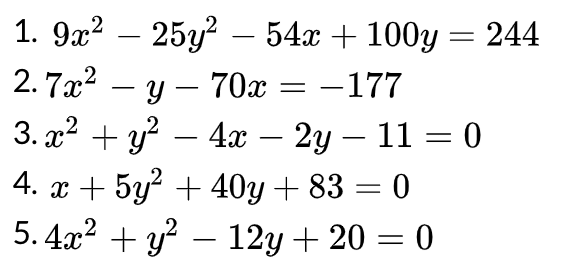# 1. 92252 - 54x + 100y = 2442. 7a2 70x = -1773. г2 + у? — 4a — 2у — 11 — 04. x5y240y83 05.4a270хy2-12y20 0

Question
21 views

For each equation, decide which type of conic section each equation represents (parabola, ellipse, hyperbola, circle).  If it is none of them, state that as well.  You must show work for this and/or explain how you can identify each equation.help_outlineImage Transcriptionclose1. 92252 - 54x + 100y = 244 2. 7a2 70x = -177 3. г2 + у? — 4a — 2у — 11 — 0 4. x5y240y83 0 5.4a2 70х y2-12y20 0 fullscreen
check_circle

Step 1

To identify the type of conic section observe the given equation,

Circle: Both x2 and y2 is present and their coefficients must be same including the sign.

Parabola: Any one of x2 or y2 is present not both.

Ellipse: Both x2 and y2 is present and their coefficients must be different and positive

Hyperbola: Both x2 and y2 is present and one of them has positive coefficient and other one has negative coefficient.

Step 2

Observe the given equation,

Step 3

Here, both x2 and y2 is present.

Coefficient of x2h is 9( positive) and coefficient of y2 is -...

### Want to see the full answer?

See Solution

#### Want to see this answer and more?

Solutions are written by subject experts who are available 24/7. Questions are typically answered within 1 hour.*

See Solution
*Response times may vary by subject and question.
Tagged in

### Math